• 文章目录实验目的1、区域生长算法2、区域分裂合并算法3、分水岭分割算法 实验目的 编写程序完成以下算法，并进行比较，得出结论。 区域生长算法 区域分裂合并算法 分水岭分割算法 1、区域生长算法 2、区域分裂合并...
文章目录实验前言与目的1、区域生长算法注意代码2、区域分裂合并算法注意代码3、分水岭分割算法代码项目代码致谢
实验前言与目的
这些算法都属于【基于形态学的图像分割】这一范畴，我们最终做的一切，都是为了图像分割（比如分成黑白两块，相对提取物体主题，为物体描轮廓…）
编写程序完成以下算法，并进行比较，得出结论。

区域生长算法
区域分裂合并算法
分水岭分割算法

1、区域生长算法

参考：https://blog.csdn.net/weixin_43487953/article/details/97395528

注意

区域生长是一种图像分割方法。
区域生长从某个像素出发，按照一定的准则，逐步加入邻近像素，当满足一定的条件时，区域生长终止，进而实现目标的提取。
区域生长的好坏决定于：1.初始点（种子点）的选取。2.生长准则。3.终止条件。
个人感悟：这个算法平均感觉都挺慢的，我运行一个狗子的图，即使换了不同的种子，平均也在10S~20S之间。

PPT思路本程序思路:

先生成同样大小空白矩阵
设置一个种子点，通过计算判断周围是否有符合条件新种子，编写在空白矩阵中
通过循环可以获得含有种子标志的新矩阵，将矩阵与原图相乘可以得到利用区域生长分割出的图像。
备注：由于代码不长，所以思路主要用备注写代码里了、

输入图像经过区域生成算法之后的效果：代码
################################################
#################区域生成算法###################
################################################
def 区域生成法(img):
img_array = np.array(img)#图片转为数组方便操作

[m,n]=img_array.shape#返回图片的长和宽

a = np.zeros((m,n)) #建立等大小空矩阵

a[70,70]=1 #设立种子点
k = 40 #设立生长阈值

isMyArea=1
#开始循环遍历周围像素，种子长大。
while isMyArea==1:
isMyArea=0
lim = (np.cumsum(img_array*a)[-1])/(np.cumsum(a)[-1])
for i in range(2,m):
for j in range(2,n):
if a[i,j]==1:
for x in range(-1,2):
for y in range(-1,2):
if a[i+x,j+y]==0:
if (abs(img_array[i+x,j+y]-lim)<=k) :
isMyArea = 1
a[i+x,j+y]=1
print("我是区域，我正在生长...")
data = img_array*a #矩阵相乘获取生长图像的矩阵
new_img = Image.fromarray(data) #data矩阵转化为二维图片

return new_img

2、区域分裂合并算法

参考：
https://www.jianshu.com/p/fa573431ef3d个人感觉，这个分割算法有点【微积分】那种感觉了。
不用像区域生长一样还要选个“种子点”，算法比较“稳”？

算法的思想总的来说，就是：

先把图像分成4块
若这其中的一块符合分裂条件，那么这一块又分裂成4块
分裂到一定数量时，以每块为中心，检查相邻的各块，满足一定条件，就合并。
如此循环往复进行分裂和合并的操作。
最后合并小区，即把一些小块图像的合并到旁边的大块里。

注意

本次切割图像不使用专门的库，而是直接通过控制img[x,y,weight,height]来最原图片的一个个小区域直接操作（具体操作是二值化）。
从最终成像来看，区域分裂与合并算法的确具有一定的连续性，不会出现黑图中夹杂着一丝白丝儿的那种噪声。

效果图代码
import numpy as np
import cv2
import matplotlib.pyplot as plt # plt 用于显示图片

#判断方框是否需要再次拆分为四个
def judge(w0, h0, w, h):
a = img[h0: h0 + h, w0: w0 + w]
ave = np.mean(a)
std = np.std(a, ddof=1)
count = 0
total = 0
for i in range(w0, w0 + w):
for j in range(h0, h0 + h):
#注意！我输入的图片数灰度图，所以直接用的img[j,i]，RGB图像的话每个img像素是一个三维向量，不能直接与avg进行比较大小。
if abs(img[j, i] - ave) < 1 * std:
count += 1
total += 1
if (count / total) < 0.95:#合适的点还是比较少，接着拆
return True
else:
return False

##将图像将根据阈值二值化处理，在此默认125
def draw(w0, h0, w, h):
for i in range(w0, w0 + w):
for j in range(h0, h0 + h):
if img[j, i] > 125:
img[j, i] = 255
else:
img[j, i] = 0

def function(w0, h0, w, h):
if judge(w0, h0, w, h) and (min(w, h) > 5):
function(w0, h0, int(w / 2), int(h / 2))
function(w0 + int(w / 2), h0, int(w / 2), int(h / 2))
function(w0, h0 + int(h / 2), int(w / 2), int(h / 2))
function(w0 + int(w / 2), h0 + int(h / 2), int(w / 2), int(h / 2))
else:
draw(w0, h0, w, h)

##############################################
######################main_##################
###############################################

height, width = img.shape

function(0, 0, width, height)

cv2.imshow('input',img_input)
cv2.imshow('output',img)

cv2.waitKey()
cv2.destroyAllWindows()


3、分水岭分割算法

参考opencv4  python 中文文档

思路：

分水岭算法实际上是根据图像的灰度值和形态学知识（膨胀啊，腐蚀啊），用一种标记来确定对象区域。
用另一种标记确定非对象的区域。
最后用 0 标记我们不确定的区域。
然后应用分水岭算法。然后我们的标记将使用我们给出的标签进行更新，对象的边界值将为 -1 。

实现：由于这部分代码有点长，所以将分步展开讲

先做图像的预处理，包括二值化和去噪（不去噪会导致图像的过度分割）2. 在图像划分出对象区域和非对象区域，并且通过二者相减，获得不确定区域：3. 为区域做标记：4. 执行分水岭算法，将分水岭画成明显的线打到图上：效果图代码
################################################
##################分水岭########################

def 分水岭算法(img):
#转化成灰度图，方便处理
gray=cv2.cvtColor(img,cv2.COLOR_BGR2GRAY)

#二值化
ret,thresh=cv2.threshold(gray,0,255,cv2.THRESH_BINARY_INV+cv2.THRESH_OTSU)

#去除噪音(要不然最终成像会导致过度分割)
kernel=np.ones((3,3),np.uint8)
opening=cv2.morphologyEx(thresh,cv2.MORPH_OPEN,kernel,iterations=2)

#确定非对象区域
sure_bg=cv2.dilate(opening,kernel,iterations=3)#进行膨胀操作

#确定对象区域
dist_transform=cv2.distanceTransform(opening,1,5)
ret,sure_fg=cv2.threshold(dist_transform,0.7*dist_transform.max(),255,0)

#寻找未知的区域
sure_fg=np.uint8(sure_fg)
unknown=cv2.subtract(sure_bg,sure_fg)#非对象区域减去对象区域就是不确定区域

# 为对象区域类别标记
ret, markers = cv2.connectedComponents(sure_fg)
# 为所有的标记加1，保证非对象是0而不是1
markers = markers+1
# 现在让所有的未知区域为0
markers[unknown==255] = 0

#执行分水岭算法
markers = cv2.watershed(img,markers)
img[markers == -1] = [255,0,0]

####################################################
########################打印########################

#解决中文显示问题
plt.rcParams['font.sans-serif']=['SimHei']
plt.rcParams['axes.unicode_minus'] = False

plt.subplot(231), plt.imshow(gray, 'gray'), plt.title('输入图片'),plt.axis('off')
plt.subplot(232), plt.imshow(opening,'gray'), plt.title('二值化去噪之后'),plt.axis('off')
plt.subplot(233), plt.imshow(sure_bg,'gray'), plt.title('确定非对象区域'),plt.axis('off')
plt.subplot(234), plt.imshow(dist_transform,'gray'), plt.title('确定对象区域'),plt.axis('off')
plt.subplot(235), plt.imshow(unknown,'gray'), plt.title('未知区域'),plt.axis('off')
plt.subplot(236), plt.imshow(img,'gray'), plt.title('分水岭算法'),plt.axis('off')

plt.show()

项目代码
import cv2
from PIL import Image
import matplotlib.pyplot as plt # plt 用于显示图片
import numpy as np

################################################
#################区域生成算法###################
################################################

def 区域生成法(img):
img_array = np.array(img)#图片转为数组方便操作

[m,n]=img_array.shape#返回图片的长和宽

a = np.zeros((m,n)) #建立等大小空矩阵

a[100,100]=1 #设立种子点
k = 30 #设立生长阈值，灰度小于此值，则纳入区域。

isMyArea=1
while isMyArea==1:
isMyArea=0
lim = (np.cumsum(img_array*a)[-1])/(np.cumsum(a)[-1])
for i in range(2,m):
for j in range(2,n):
if a[i,j]==1:
for x in range(-1,2):
for y in range(-1,2):
if a[i+x,j+y]==0:
if (abs(img_array[i+x,j+y]-lim)<=k) :
isMyArea = 1
a[i+x,j+y]=1
print("我是区域，我正在生长...")
data = img_array*a #矩阵相乘获取生长图像的矩阵
new_img = Image.fromarray(data) #data矩阵转化为二维图片

return new_img

################################################
################区域分裂与合并###################
##################################################

import numpy as np
import cv2
import matplotlib.pyplot as plt # plt 用于显示图片

#判断方框是否需要再次拆分为四个
def judge(w0, h0, w, h):
a = img[h0: h0 + h, w0: w0 + w]
ave = np.mean(a)
std = np.std(a, ddof=1)
count = 0
total = 0
for i in range(w0, w0 + w):
for j in range(h0, h0 + h):
if abs(img[j, i] - ave) < 1 * std:
count += 1
total += 1
if (count / total) < 0.95:
return True
else:
return False

##将图像将根据阈值二值化处理，在此默认125
def draw(w0, h0, w, h):
for i in range(w0, w0 + w):
for j in range(h0, h0 + h):
if img[j, i] > 125:
img[j, i] = 255
else:
img[j, i] = 0

def function(w0, h0, w, h):
if judge(w0, h0, w, h) and (min(w, h) > 5):
function(w0, h0, int(w / 2), int(h / 2))
function(w0 + int(w / 2), h0, int(w / 2), int(h / 2))
function(w0, h0 + int(h / 2), int(w / 2), int(h / 2))
function(w0 + int(w / 2), h0 + int(h / 2), int(w / 2), int(h / 2))
else:
draw(w0, h0, w, h)

################################################
##################分水岭########################
################################################

def 分水岭算法(img):
#转化成灰度图，方便处理
gray=cv2.cvtColor(img,cv2.COLOR_BGR2GRAY)

#二值化
ret,thresh=cv2.threshold(gray,0,255,cv2.THRESH_BINARY_INV+cv2.THRESH_OTSU)

#去除噪音(要不然最终成像会导致过度分割)
kernel=np.ones((3,3),np.uint8)
opening=cv2.morphologyEx(thresh,cv2.MORPH_OPEN,kernel,iterations=2)

#确定非对象区域
sure_bg=cv2.dilate(opening,kernel,iterations=3)#进行膨胀操作

#确定对象区域
dist_transform=cv2.distanceTransform(opening,1,5)
ret,sure_fg=cv2.threshold(dist_transform,0.7*dist_transform.max(),255,0)

#寻找未知的区域
sure_fg=np.uint8(sure_fg)
unknown=cv2.subtract(sure_bg,sure_fg)#非对象区域减去对象区域就是不确定区域

# 为对象区域类别标记
ret, markers = cv2.connectedComponents(sure_fg)
# 为所有的标记加1，保证非对象是0而不是1
markers = markers+1
# 现在让所有的未知区域为0
markers[unknown==255] = 0

#执行分水岭算法
markers = cv2.watershed(img,markers)
img[markers == -1] = [255,0,0]

#解决中文显示问题
plt.rcParams['font.sans-serif']=['SimHei']
plt.rcParams['axes.unicode_minus'] = False

plt.subplot(231), plt.imshow(gray, 'gray'), plt.title('输入图片'),plt.axis('off')
plt.subplot(232), plt.imshow(opening,'gray'), plt.title('二值化去噪之后'),plt.axis('off')
plt.subplot(233), plt.imshow(sure_bg,'gray'), plt.title('确定非对象区域'),plt.axis('off')
plt.subplot(234), plt.imshow(dist_transform,'gray'), plt.title('确定对象区域'),plt.axis('off')
plt.subplot(235), plt.imshow(unknown,'gray'), plt.title('未知区域'),plt.axis('off')
plt.subplot(236), plt.imshow(img,'gray'), plt.title('分水岭算法'),plt.axis('off')

plt.show()

###################################################
####################主函数#########################

#区域生成法
#result1 = 区域生成法(img)

#区域分裂合并
height, width = img.shape

function(0, 0, width, height)

cv2.imshow('input',img_input)
cv2.imshow('output',img)
cv2.waitKey()
cv2.destroyAllWindows()

#分水岭算法
#result3 = 分水岭算法(img3)

####################################################
########################打印########################

#解决中文显示问题
plt.rcParams['font.sans-serif']=['SimHei']
plt.rcParams['axes.unicode_minus'] = False

#plt.subplot(221), plt.imshow(img, 'gray'), plt.title('输入图片'),plt.axis('off')
#plt.subplot(222), plt.imshow(pic1,'gray'), plt.title('2'),plt.axis('off')
#plt.subplot(222), plt.imshow(pic2,'gray'), plt.title('3'),plt.axis('off')
#plt.subplot(222), plt.imshow(pic3,'gray'), plt.title('4'),plt.axis('off')

#plt.show()

致谢
最后，感谢舍友再区域分裂与合并算法中的智力支持


展开全文区域生长 数字图像处理
• python版本的GrabCut前景分割和分水岭分割，同时也有深度估计
• ## Python之opencv 分水岭分割算法

千次阅读 多人点赞 2017-07-17 17:16:53
分水岭分割算法 分水岭分割算法的定义网上随便百度一下就可以知道了，我就说一下我的理解，有不对的希望大家也可以帮忙指正。 对于分水岭分割，我的理解是，一幅图像是由不同大小的灰度级像素值构成的，可以把不同...
分水岭分割算法
分水岭分割算法的定义网上随便百度一下就可以知道了，我就说一下我的理解，有不对的希望大家也可以帮忙指正。
对于分水岭分割，我的理解是，一幅图像是由不同大小的灰度级像素值构成的，可以把不同的大小想象成不同高度的山脉，接着在地表（就是从像素灰度级0开始）向这个山脉地脉注入水，那么当一个山脉与另一个山脉将要融合的线上就是图像的边界，当水注入最高山脉后形成的现象就是整幅图的边界。

cv2.watershed(参数1，参数2)

Python中的分水岭分割算法有两个参数，第一个参数是带分割的图像，且为8位三通道彩色图像，第二个参数的掩码，第二个参数必须是32位单通道的图像。下面是完整代码，在使用分水岭分割算法之前的步骤是为了获取掩码图像

import cv2
import numpy as np
cv2.imshow('img',img)
#将图像转化为灰度图像
gray=cv2.cvtColor(img,cv2.COLOR_BGR2GRAY)
#阈值化处理
ret,thresh=cv2.threshold(gray,0,255,cv2.THRESH_BINARY_INV+cv2.THRESH_OTSU)
cv2.imshow('thresh',thresh)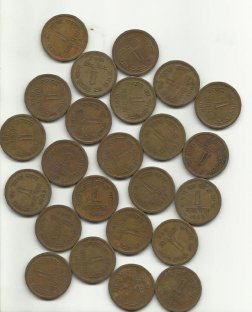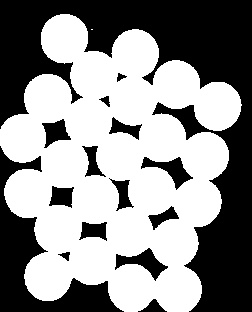#noise removal
#opening operator是先腐蚀后膨胀，可以消除一些细小的边界，消除噪声
kernel=np.ones((3,3),np.uint8)
opening=cv2.morphologyEx(thresh,cv2.MORPH_OPEN,kernel,iterations=2)
cv2.imshow('opening',opening)

二值化后由于图像存在一些噪声（即白色的点），可以用形态学的开操作的处理。opening操作可以消除物体之间细小的粘缝，为了展示openin操作的效果，我将内核设置为5*5，并且迭代了5次，其效果如下：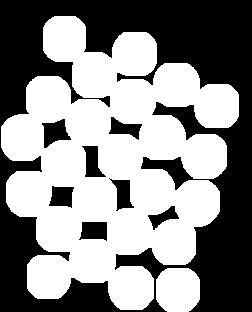获得掩码需要知道图像的前景和背景，在这里我的理解是：前景就是有硬币的图像，背景就是除硬币之前的区域。我们要确保我们获得的背景图中不包含前景图的区域，通过腐蚀操作，将硬币的区域放大，那么剩下的就一定是背景区域了，即可以确定背景图


#sure background area
sure_bg=cv2.dilate(opening,kernel,iterations=3)
cv2.imshow('bg',sure_bg)

背景图效果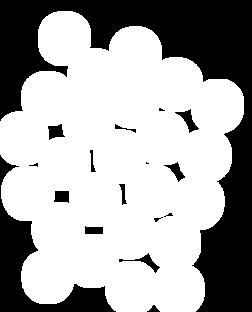如上所说，确定前景，就是硬币的区域 距离变化        可用来实现目标细化、骨架提取、形状插值及匹配、粘连物体的分离等。距离变换是针对二值图像的一种变换。在二维空间中，一幅二值图像可以认为仅仅包含目标和背景两种像素，目标的像素值为1，背景的像素值为0；距离变换的结果不是另一幅二值图像，而是一幅灰度级图像，即距离图像，图像中每个像素的灰度值为该像素与距其最近的背景像素间的距离（就是原二值图像中像素值为1的像素点与最近的像素点为0的像素点之间的距离）
由于掩码是一幅二值图像，所以经过距离变化后还需要将图像进行二值化
这样子的结果图像中像素点为1的区域就一定是硬币的位置，即前景图

#finding sure foreground area
dist_transfrom=cv2.distanceTransform(opening,cv2.DIST_L2 ,5)
#cv2.imshow('dist_transfrom',dist_transfrom)
ret,sure_fg=cv2.threshold(dist_transfrom,0.7*dist_transfrom.max(),255,0)

cv2.imshow('sure_fg',sure_fg)

前景图效果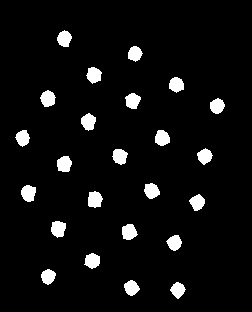现在我们已经知道原图像的前景图和背景图了，但是还有一些区域是我们所不知道的就是原图像中硬币与硬币之间相连的那些或者叠加的区域，即边界，通过背景图减去前景图可以大概的获得这些未知的边界


#finding unknow region
sure_fg=np.uint8(sure_fg)
unknow=cv2.subtract(sure_bg,sure_fg) #背景-前景
cv2.imshow('unknow',unknow)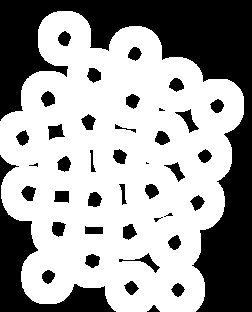官网上说connectedComponents函数可以使图像中标记背景像素点为0，飞背景像素点从1开始累加分别标记

ret,maker=cv2.connectedComponents(sure_fg)
maker=maker+1
maker[unknow==255]=0

最终使用分水岭分割算法

maker = cv2.watershed(img,maker)
cv2.imshow('maker',maker)
img[maker == -1] = [0,0,255]
cv2.imshow('result',img)

在经过分水岭分割算法后，边界处会标记为-1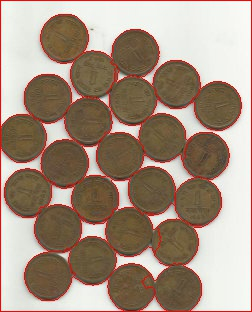这里面有几个点我还没有很清楚的理解，等后续理解了再来更新 官网参考文献
展开全文opencv
• 本文内容是对Opencv官方文档的学习笔记原理我们可以将任何一幅灰度图都看作是拓扑平面，灰度图中灰度值高的看作是山峰，灰度值地的看作是山谷...我们构建好的堤坝就是对图像的分割。但是由于噪声或者图像中其他不规...
本文内容是对Opencv官方文档的学习笔记原理我们可以将任何一幅灰度图都看作是拓扑平面，灰度图中灰度值高的看作是山峰，灰度值地的看作是山谷，我们向每一个山谷中灌入不同颜色的水，随着水位的升高，不同山谷的水就会相遇汇合，而为了防止不同山谷的水汇合，我们需要在水汇合的地方建起堤坝，随着不停地灌水，不停地构建堤坝，直到所有的山峰都被水淹没。我们构建好的堤坝就是对图像的分割。但是由于噪声或者图像中其他不规律的因素，这种方法可能会得到过度分割的效果。为减少这种影响，OpenCV采用基于掩模的分水岭算法，在这种算法中我们要设置哪些山谷点会汇合，哪些不会。这是一种交互式的图像分割。我们要做的就是给我们已知的对象打上不同的标签。如果某个区域肯定是前景或对象，就使用某个颜色(或灰度值)标签标记它。如果某个区域肯定不是对象而是背景就使用另外一个颜色标签标记。而剩下的不能确定是前景还是背景的区域就用 0 标记。这就是我们的标签。然后实施分水岭算法。每一次灌水，我们的标签就会被更新，当两个不同颜色的标签相遇时就构建堤坝，直到将所有山峰淹没，最后我们得到的边界对象(堤坝)的值为 -1。代码示例在下面的例子中，我们将距离变换和分水岭算法紧挨在一起的对象进行分割。看一下原图：original先从硬币的近似估值开始，我们先使用二值化cv2.threshold函数对图像进行处理import numpy as npimport cv2from matplotlib import pyplot as pltimg = cv2.imread('water_coins.jpg')gray=cv2.cvtColor(img,cv2.COLOR_BGR2GRAY)#转化成灰度图ret,thresh=cv2.threshold(gray,0,255,cv2.THRESH_BINARY_INV+cv2.THRESH_OTSU)#二值化#看一下图像cv2.imshow('orig',img)cv2.imshow('thresh',thresh)cv2.waitKey(0)cv2.destroyAllWindows()１现在我们开运算去除图像中的所有白噪声。靠近对象中心的区域肯定是前景，而远离对象中心的区域肯定是背景，不能确定的部分为硬币之间的边界。我们要提取肯定是硬币的区域，距离变换+合适的阈值。接下来我们要找肯定不是硬币的区域，用膨胀操作，膨胀操作将对象的边界延伸到了背景中，所以由于边界区域被处理，我们就能知道哪些区域是前景，哪些区域是背景。剩下的区域就是我们不知道该如何区分了，而这就是分水岭算法要做的，前景与背景的交界处就是边界，从肯定是不是背景的区域中减去肯定是前景的区域就是边界区域。#去除噪音kernel=np.ones((3,3),np.uint8)opening=cv2.morphologyEx(thresh,cv2.MORPH_OPEN,kernel,iterations=2)#确定背景区域sure_bg=cv2.dilate(opening,kernel,iterations=3)#确定前景区域dist_transform=cv2.distanceTransform(opening,1,5)ret,sure_fg=cv2.threshold(dist_transform,0.7*dist_transform.max(),255,0)#寻找未知的区域sure_fg=np.uint8(sure_fg)unknown=cv2.subtract(sure_bg,sure_fg)cv2.imshow('sure_bg',sure_bg)cv2.imshow('sure_fg',sure_fg)cv2.imshow('unknow',unknow)cv2.waitKey(0)cv2.destroyAllWindows()2在上面的代码中，阈值化以后得到的就是硬币的区域。现在知道了哪些是背景，哪些是硬币，我们就可以创建标签了(一个与原图像大小相同，数据类型为int32的数组)并标记其中的区域，用函数 cv2.connectedComponents()来操作，将背景标记为0，其他对象使用从１开始的正整数标记。对不确定的区域(函数cv2.connectedComponents()输出的结果使用unknown定义未知区域)标记为０。# Marker labellingret,markers1=cv2.connectedComponents(sure_fg)# Add one to all labels so that sure background is not 0, but 1markers = markers1+1# Now, mark the region of unknown with zeromarkers[unknown==255] = 0深蓝色区域为未知区域。肯定是硬币的区域使用不同的颜色标记。其余区域就是用浅蓝色标记的背景了。现在标签准备好了。到最后一步：实施分水岭算法了。标签图像将会被修改，边界区域的标记将变为 -1.markers3 = cv2.watershed(img,markers)img[markers3 == -1] = [255,0,0]cv2.imshow('img',img)cv2.waitKey(0)cv2.destroyAllWindows()结果如下。有些硬币的边界被分割的很好，也有一些硬币之间的边界分割的不好。3
展开全文• 直接上代码，原理大家都可以查到 注意：以下img自己加载即可，我是事先把图片转换成了单通道的Array形式，并且我的img是归一化后在0-1的。 import cv2 import numpy as np import copy imgc = copy.deepcopy(img) ...
直接上代码，原理大家都可以查到
注意：以下img自己加载即可，我是事先把图片转换成了单通道的Array形式，并且我的img是归一化后在0-1的。
import cv2
import numpy as np
import copy
def watershed(img):

gray = img
ret0, thresh0 = cv2.threshold(gray,0,255,cv2.THRESH_BINARY_INV+cv2.THRESH_OTSU)

kernel = np.ones((3,3),np.uint8)
opening = cv2.morphologyEx(thresh0,cv2.MORPH_OPEN,kernel, iterations = 2)

# 确定背景区域
sure_bg = cv2.dilate(opening,kernel,iterations=3)

# 确定前景区域
dist_transform = cv2.distanceTransform(opening,cv2.DIST_L2,5)
ret1, sure_fg = cv2.threshold(dist_transform,0.7*dist_transform.max(),255,0)

# 查找未知区域
sure_fg = np.uint8(sure_fg)
unknown = cv2.subtract(sure_bg,sure_fg)

# 标记标签
ret2, markers1 = cv2.connectedComponents(sure_fg)
markers = markers1+1
markers[unknown==255] = 0

markers3 = cv2.watershed(img,markers)
img[markers3 == -1] = [0,255,0]
return thresh0,sure_bg,sure_fg,img

imgc = copy.deepcopy(img)
gray = copy.deepcopy(img)
ret, thresh = cv.threshold(gray, 0, 255, cv.THRESH_BINARY_INV + cv.THRESH_OTSU)
# noise removal
kernel = np.ones((3, 3), np.uint8)
opening = cv2.morphologyEx(thresh, cv2.MORPH_OPEN, kernel, iterations=2)

# sure background area
sure_bg = cv2.dilate(opening, kernel, iterations=3)

# Finding sure foreground area
# 距离变换的基本含义是计算一个图像中非零像素点到最近的零像素点的距离，也就是到零像素点的最短距离
# 最常见的距离变换算法就是通过连续的腐蚀操作来实现，腐蚀操作的停止条件是所有前景像素都被完全
# 腐蚀。这样根据腐蚀的先后顺序，我们就得到各个前景像素点到前景中心骨架像素点的距离。
# 根据各个像素点的距离值，设置为不同的灰度值。这样就完成了二值图像的距离变换
# 第二个参数 0,1,2 分别表示 CV_DIST_L1, CV_DIST_L2 , CV_DIST_C
dist_transform = cv2.distanceTransform(opening, 1, 5)
ret, sure_fg = cv2.threshold(dist_transform, 0.7 * dist_transform.max(), 255, 0)

# Finding unknown region
sure_fg = np.uint8(sure_fg)
unknown = cv2.subtract(sure_bg, sure_fg)
# Marker labelling
ret, markers = cv.connectedComponents(sure_fg)

# Add one to all labels so that sure background is not 0, but 1
markers = markers + 1

# Now, mark the region of unknown with zero
markers[unknown == 255] = 0

imgc = cv.cvtColor(img, cv.COLOR_GRAY2BGR)
markers = cv.watershed(imgc, markers)

imgc[markers == -1] = [255,0,0]
label = imgc



展开全文• 通过分水岭算法分割细胞图像的python实现，可以直接使用，需要安装有openCV、mahotas、numpy、matplotlib包，然后python的版本最好是3.x，容易看不懂的地方都加了注释。
• 分水岭分割流程：图像-&gt;灰度-&gt;二值-&gt;距离变换-&gt;寻找种子-&gt;生成Marker-&gt;分水岭变换-&gt;输出具体代码如下：# -*- coding=GBK -*- import cv2 as cv import numpy as ...
• 分水岭算法分割 处理图片:[my_coin.jpg] import cv2 import matplotlib.pyplot as plt import numpy as np def main(): # 1.导入图片 img_src = cv2.imread("my_coin.jpg") # 2.转换为灰度图片 img_gray = ...
• 分水岭分割方法，是一种基于拓扑理论的数学形态学的分割方法，其基本思想是把图像看作是测地学上的拓扑地貌，图像中每一点像素的灰度值表示该点的海拔高度，每一个局部极小值及其影响区域称为集水盆，而集水盆的边界...OpenCV
• 文章目录一、前言二、cv2.distanceTransform(src, distanceType, maskSize)三、基于标记的分水岭分割功能四、示例代码 一、前言 分水岭算法是一种图像区域分割法，在分割的过程中，它会把跟临近像素间的相似性作为...
• 为避免这种情况，需要在水合并的位置建立障碍，在所有的山峰都被水淹没之前，要继续填满水和建造栅栏的工作然后你创建的障碍会给你分割的结果，这就是分水岭背后的“哲学”.可以访问我了解更多相关内容.这种方法会...
• 分水岭算法-源码（python-opencv）。
• 分水岭算法用于分割多个相邻的物体。原理灰度图像根据灰度值可以把像素之间的关系看成山峰和山谷的关系，高亮度（灰度值高）的地方是山峰，低亮度的地方是山谷。给每个孤立的山谷（局部最小值）不同颜色的水（label...
• 为避免这种情况，需要在水合并的位置建立障碍，在所有的山峰都被水淹没之前，要继续填满水和建造栅栏的工作然后你创建的障碍会给你分割的结果，这就是分水岭背后的“哲学”.可以访问我了解更多相关内容.这种方法会...
• # -*- coding: utf-8 -*- """ 图像分水岭分割图像 分水岭算法可以参考：http://baike.baidu.com/item/%E5%88%86%E6%B0%B4%E5%B2%AD%E7%AE%97%E6%B3%95 """ import numpy as np ......

# 分水岭分割pythonpython 订阅